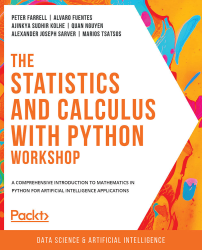•#### The Statistics and Calculus with Python Workshop#### Overview of this book

Are you looking to start developing artificial intelligence applications? Do you need a refresher on key mathematical concepts? Full of engaging practical exercises, The Statistics and Calculus with Python Workshop will show you how to apply your understanding of advanced mathematics in the context of Python. The book begins by giving you a high-level overview of the libraries you'll use while performing statistics with Python. As you progress, you'll perform various mathematical tasks using the Python programming language, such as solving algebraic functions with Python starting with basic functions, and then working through transformations and solving equations. Later chapters in the book will cover statistics and calculus concepts and how to use them to solve problems and gain useful insights. Finally, you'll study differential equations with an emphasis on numerical methods and learn about algorithms that directly calculate values of functions. By the end of this book, you’ll have learned how to apply essential statistics and calculus concepts to develop robust Python applications that solve business challenges.
Preface1. Fundamentals of PythonFree Chapter
2. Python's Main Tools for Statistics3. Python's Statistical Toolbox4. Functions and Algebra with Python5. More Mathematics with Python6. Matrices and Markov Chains with Python7. Doing Basic Statistics with Python8. Foundational Probability Concepts and Their Applications9. Intermediate Statistics with Python10. Foundational Calculus with Python11. More Calculus with Python12. Intermediate Calculus with PythonAppendix# Functions

As previously explained, functions are mathematical objects that generally take in some input and produce a desired output. A function is therefore often considered as a mapping of one mathematical object to another. When a function receives an input and subsequently produces an output, the concept of relation can also be used, which emphasizes the relationship between the set of possible inputs and that of possible outputs that is established by the function itself.

A function is typically denoted by the lowercase letter f with parentheses, which surround an input that f takes in. This symbol, f(x), also denotes the output that f produces when taking in x as input. For example, let's say the function f that outputs the square of its input; f can be denoted as f(x) = x2.

We see that the syntax for declaring a function in Python also follows this convention. For example, to declare the same squaring function in Python, the code would look like the following:

...